# Catch up on the latest AI articles# Generating High-precision Images From Limited Data With GAN

3 main points
✔️ A novel augmentation method called Adaptive Pseudo Augmentation (APA) is proposed to learn GANs with limited data.
✔️ Theoretically proved that APA converges to the optimal solution
✔️ For multiple datasets, the model using APA outperformed the traditional SOTA model

written by Liming JiangBo DaiWayne WuChen Change Loy
(Submitted on 12 Nov 2021)
NeurIPS 2021
Subjects: Computer Vision and Pattern Recognition (cs.CV); Machine Learning (cs.LG)code：The images used in this article are from the paper, the introductory slides, or were created based on them.

## first of all

While SOTA models of GANs, such as StyleGAN2, can generate very accurate images, they require a large amount of training data. However, there are cases where sufficient training data is not available due to data scarcity or privacy issues. In general, when the training data of GAN is small, the Discriminator overfits and the output distributions for true and false images are far apart. As a result, the feedback to the generator becomes meaningless information, and the accuracy of the generated image deteriorates. Therefore, in this paper, we propose that we developed a novel augmentation method called Adaptive Pseudo Augmentation (APA), which considers the generated image of the generator as a true image according to the degree of overfitting, and adaptively tricks the Discriminator to increase the accuracy. In APA, the image generated by the generator is regarded as the true image according to the overfitting degree, and the accuracy is reduced by adaptively tricking the discriminator. As a result, the Discriminator is less likely to overfit and the accuracy of the generated image can be improved.

## APA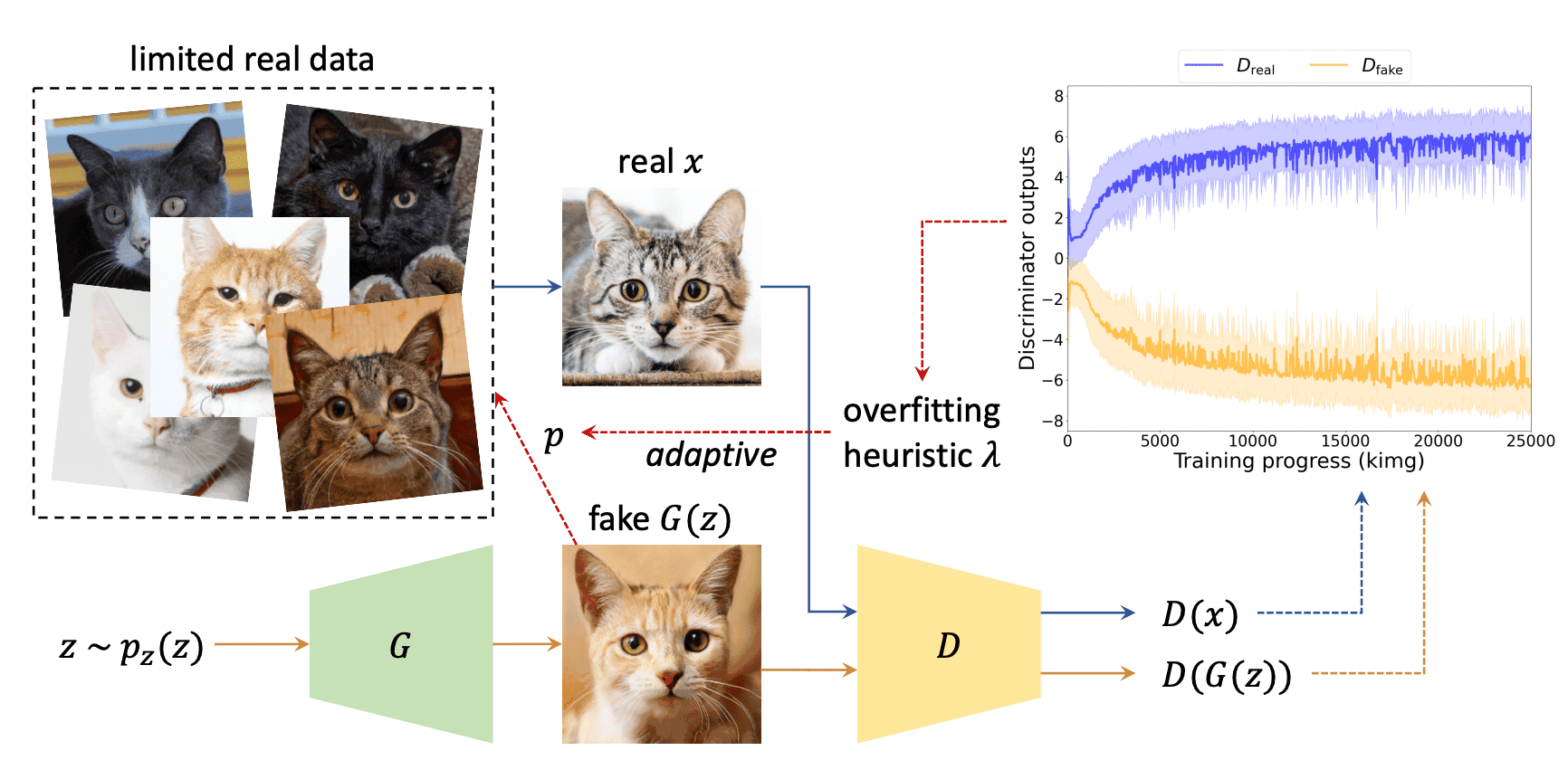As shown in the figure above, we consider the image generated by the Generator as a pseudo-true image and fool the Discriminator. Since it does not work well to treat a false image as a true image, we used a pseudo augmentation which runs with a certain probability $p \in [0,1)$ and does nothing with a probability $1-p$. Here $p$ varies with the overfit degree of the Discriminator and is adjusted using the parameter $\lambda$ defined as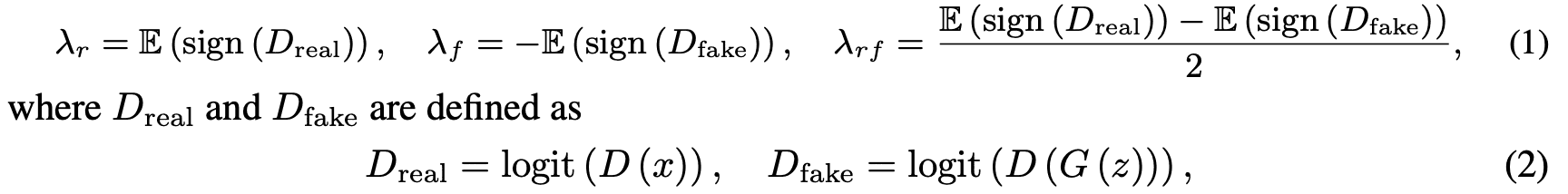where $D, G$ is the Discriminator and Generator, $x$ is the true image, $z$ is the noise parameter, and logit is the logit function. $\lambda_r$ represents the percentage of positive logit predicted by the Discriminator for the true image, and $\lambda_f$ represents the percentage for the false image. Additionally, $\lambda_{rf}$ represents the half distance between logit for the true and false images. For all $\lambda$, $\lambda=0$ represents no overfitting at all, and$\lambda=1$ represents complete overfitting. In this paper, we specifically used $\lambda_r$ as a parameter. Specifically, we initialize it at $p=0$, and for a threshold $t$(the value we mainly used was 0.6), if $\lambda$ exceeds (falls below) $t$, we increase (decrease) $p$ by one step. This can be repeated every 4 iterations, and pseudo augmentation can be done adaptively depending on the degree of overfitting.

## theoretical consideration

Let $\alpha$ be the expected value of $p$, then $0\leq\alpha<p_{\rm max}<1$ is satisfied and the value function $V(G,D)$ of Generator and Discriminator is as follows.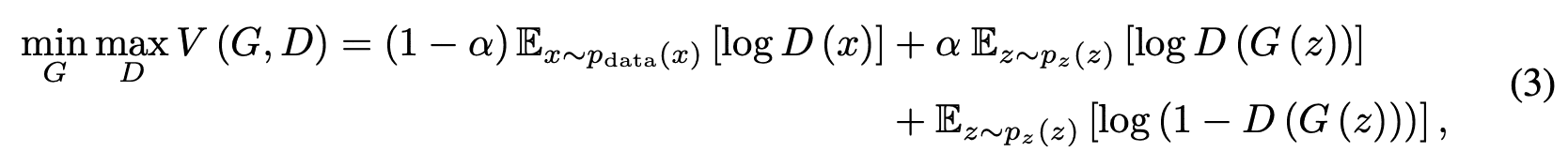Then the following proposition holds.

### Proposition 1

When $G$ is fixed, the optimal solution for $D$ is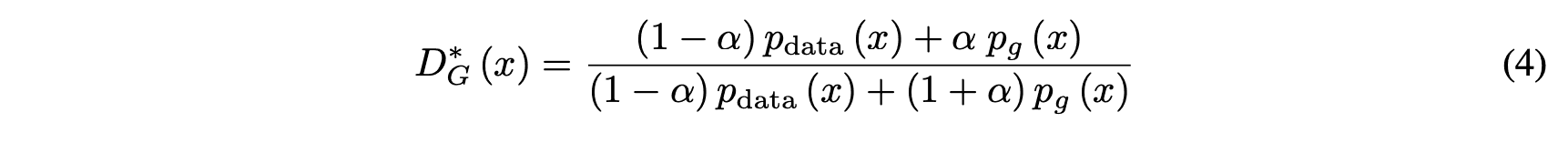where $p_{data}(x), p_g(x)$ is the probability distribution followed by true and false data

### proof

For any $G$, $D$ since the goal is to maximize $V(G,D)$.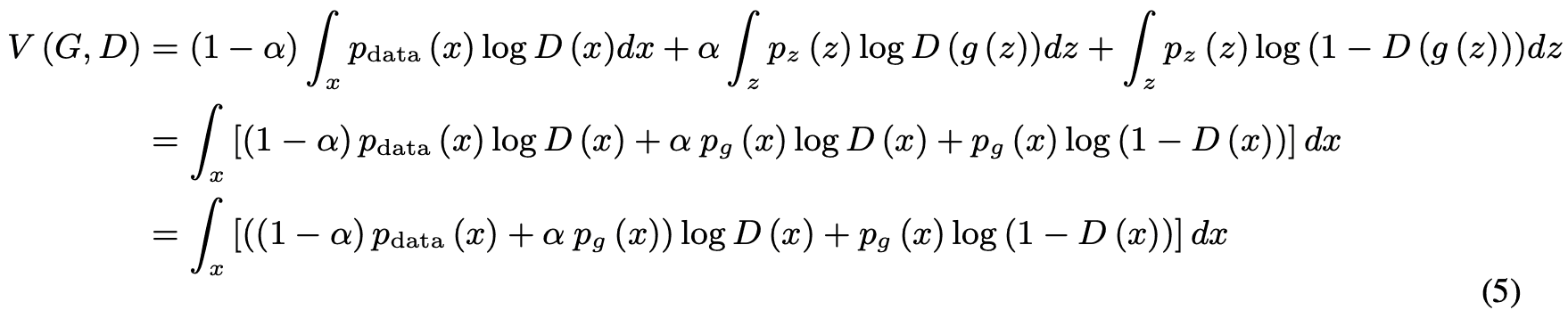Here, $f(y)=m\log(y)+n\log(1-y)$ is maximal when $y=\frac{m}{m+n}$ for any real number $(m,n)$ except $m=n=0$. Also, since $D$ is defined within the $supp$(table) of $p_{data}$ or $p_g$, Proposition 1 is satisfied. (End of proof)

By the way, when $D$ takes the optimal solution, the objective of $G$ is to minimize $V(G, D)$, but the objective of $D$ is to maximize the log-likelihood of the conditional probability $P(Y=y|x)\ (where y=0\ {\rm or}\ 1)$ of the prediction $Y$ for the input $x$, so we can write the evaluation function as We can write the evaluation function as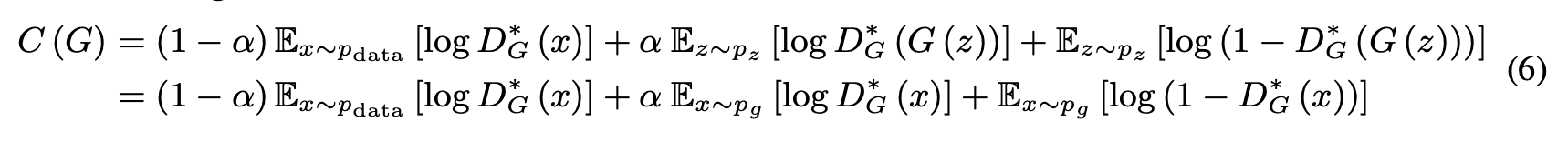Next, we consider the global minimum of $C(G)$.

### Proposition 2

$C(G)$ is a global minimum if and only if $p_g=p_{data}$, and the minimum is $C(G)=-\log 4$.

### proof

1) When $p_g=p_{data}$, from equation (4), $D^*_G(x)=\frac{1}{2}$.

Substituting this into equation (6), we get $C^*(G)=(1-\alpha)log\frac{1}{2}+\alpha\log{1}{2}+\log{1}{2}=-\log4$

2) From equation (5)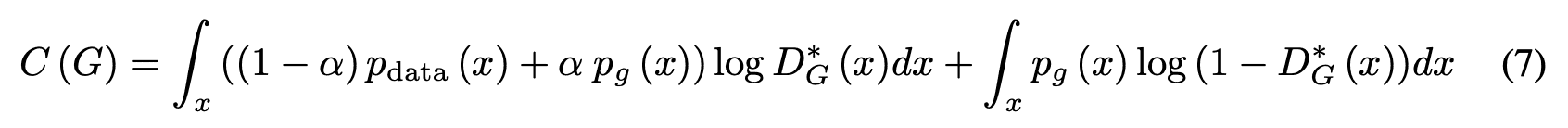Equation (6) is when $p_g=p_{data}$.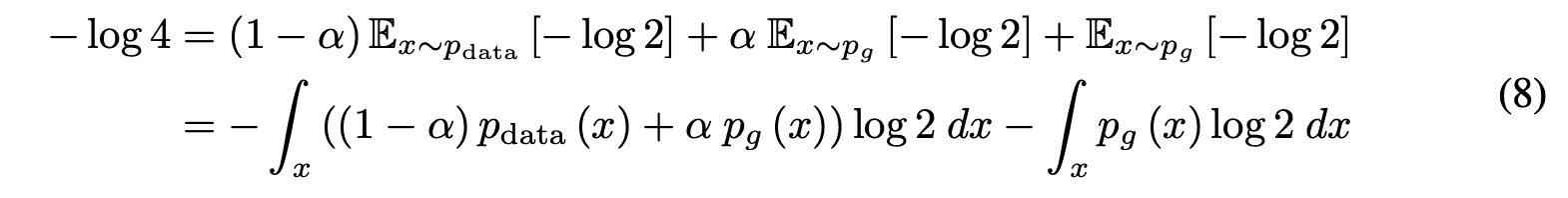Subtracting equation (7) from equation (8), we get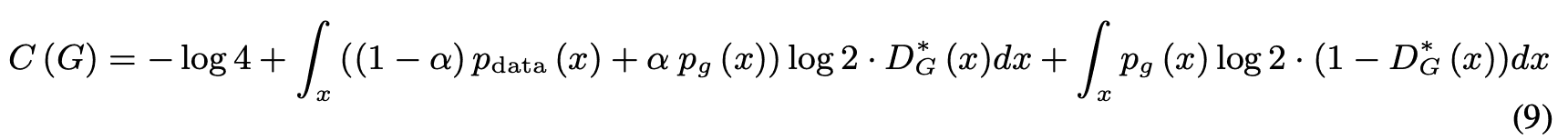Substituting equation (4) into equation (9), we get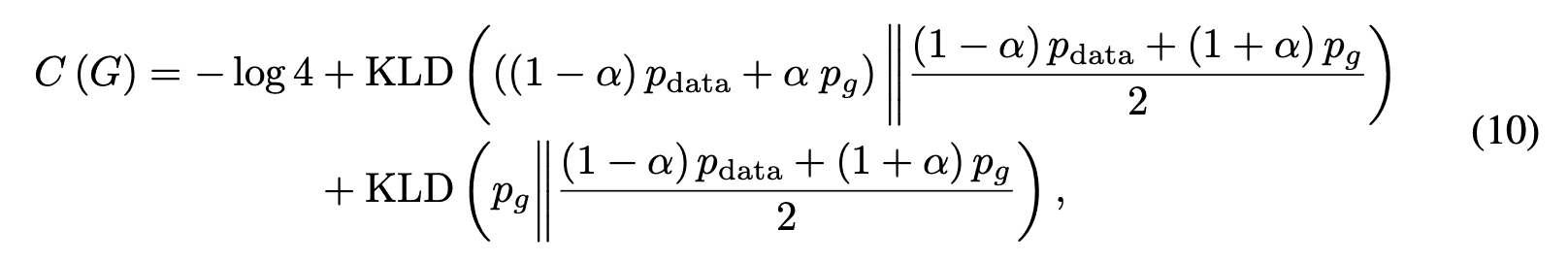Here, KLD is the KL divergence. Furthermore, equation (10) can be expressed using the JS divergence JSD as follows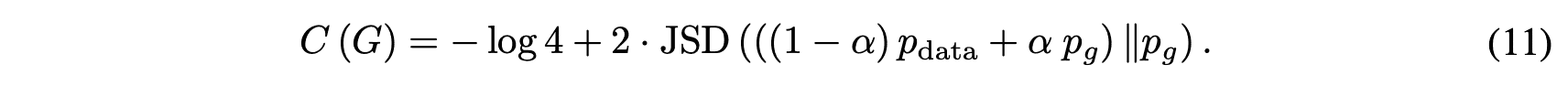Since $JSD(P||Q)$ takes the minimum value 0 when $P=Q$, $C(G)$ takes the minimum value $-\log 4$ only when ${p_g}=p_{data}$. (End of proof)

## experimental results

Trained on a limited amount of data, The images generated by StyleGAN2 and APA are shown below. For all data sets, StyleGAN2 shows degradation, while APA shows almost no degradation.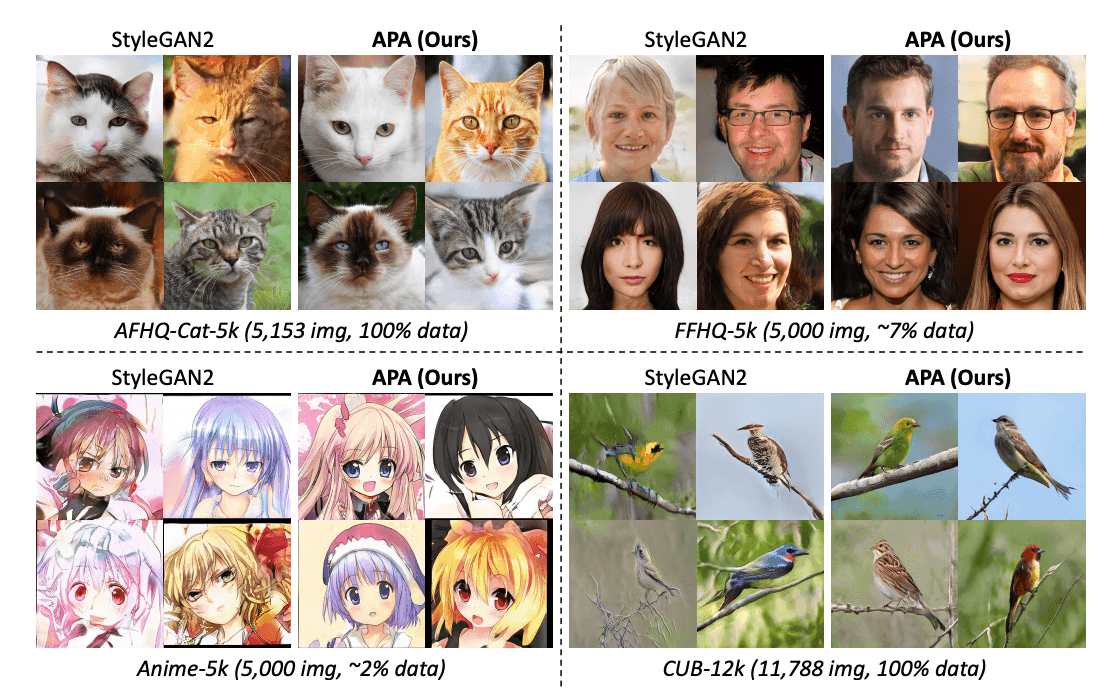The evaluation metrics used were FID (Frechet Inception Distance) and IS (Inception Score), as shown in the table below; the lower the FID, the better, and the higher the IS, the higher the performance of APA on all data sets.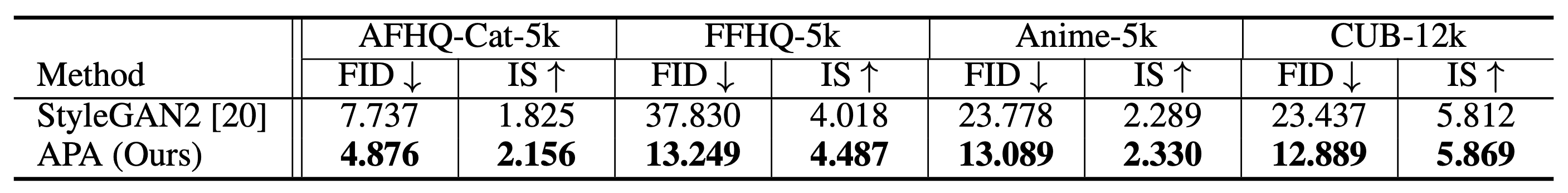Furthermore, for the FFHQ dataset, the results for varying the number of training data are shown in the figure and table below, respectively. As expected, APA outperforms StyleGAN2 qualitatively and quantitatively in all patterns, indicating that APA is effective even when trained on enough data.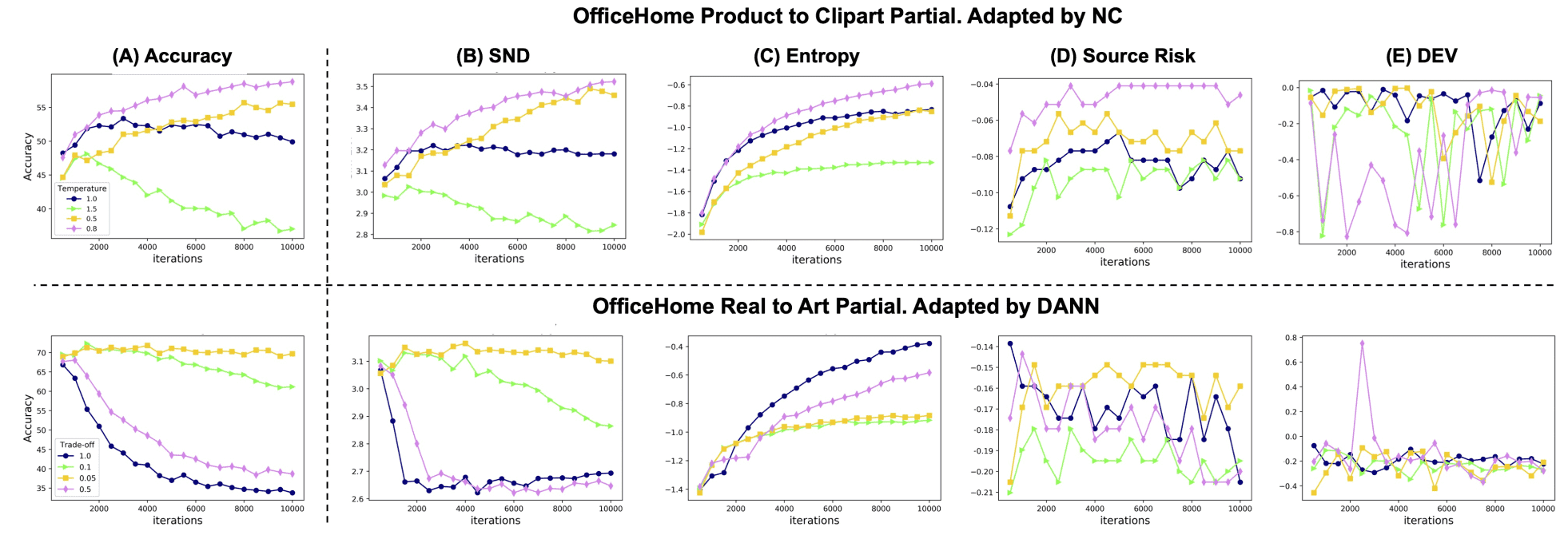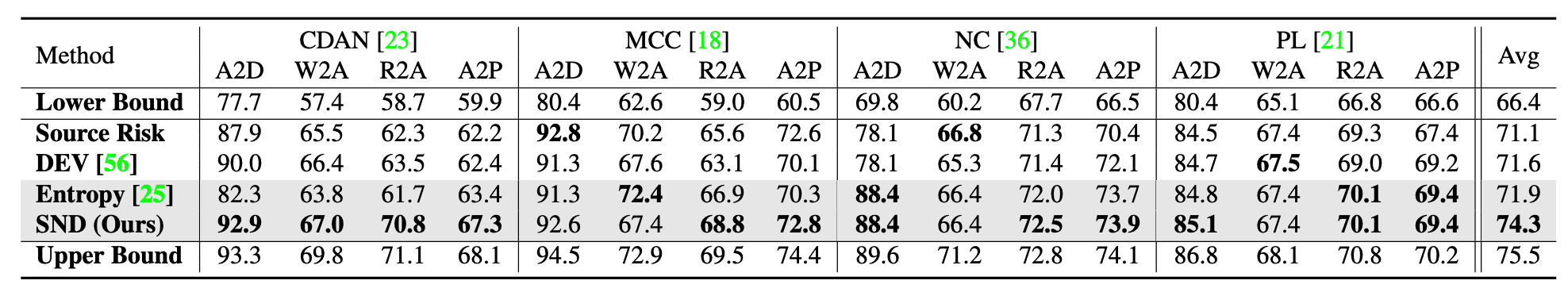As for the output of the Discriminator, in the case of StyleGAN2, the difference between true and false prediction probabilities widens when the number of data becomes small, as shown in (a) below, and it becomes overfit, while the difference of APA shrinks as shown in (b) below, and it behaves similarly to StyleGAN2 trained with sufficient amount of data. As for FID, APA converges even with limited training data, as shown in (c) below.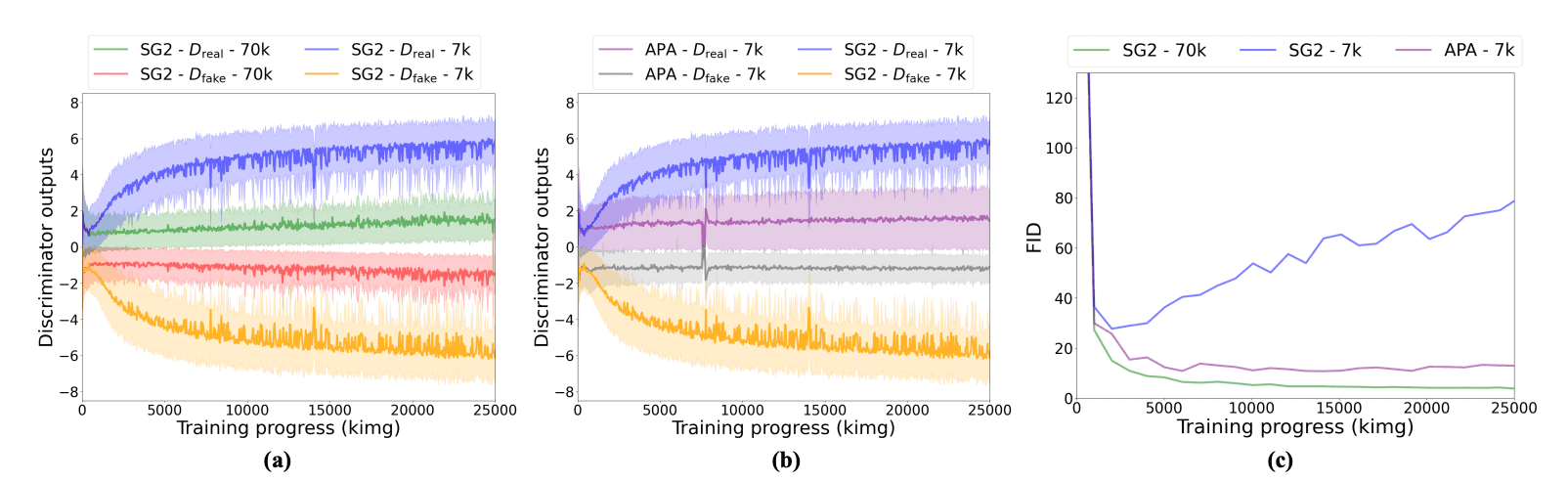Finally, the comparison results for different parameters $\lambda, p$, for both true and false label reversal, and different threshold $t$ are shown in the table below; the performance is close to the values used in APA's main, and better than StyleGAN2.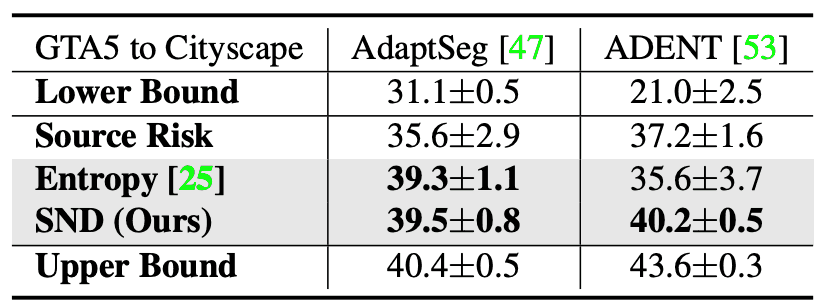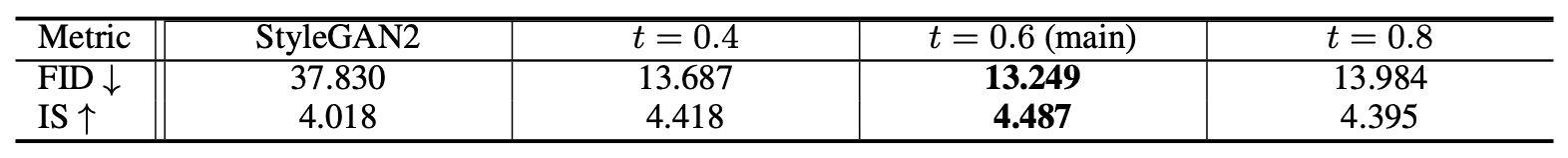## summary

In this paper, we have proposed a method called APA to generate highly accurate images in GAN from limited data. The result is a significant improvement over conventional methods with negligible computational cost and is expected to be applied in various situations in the future. However, the technique which can generate highly accurate images from limited data has the risk of being misused, and care should be taken in handling the data set.

If you have any suggestions for improvement of the content of the article,